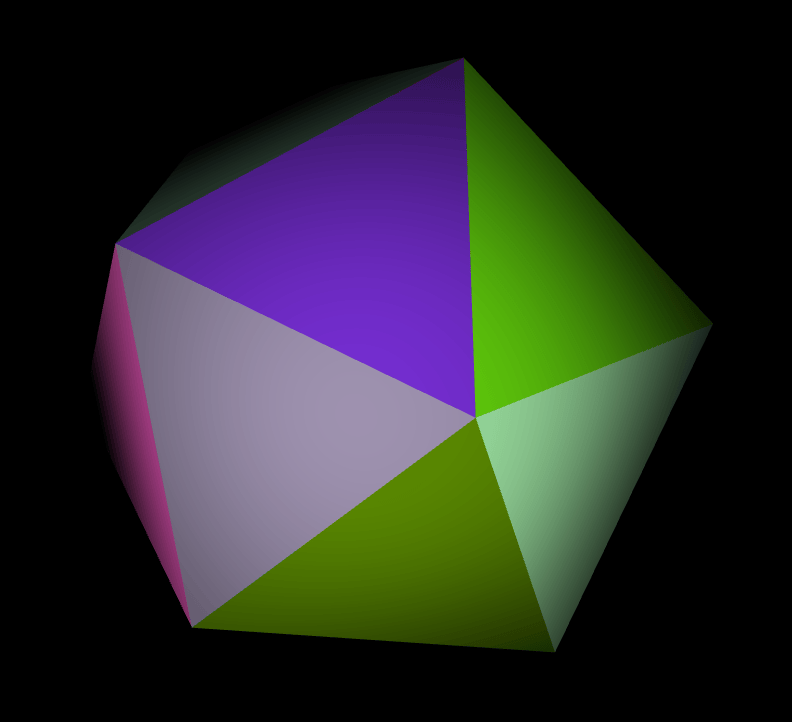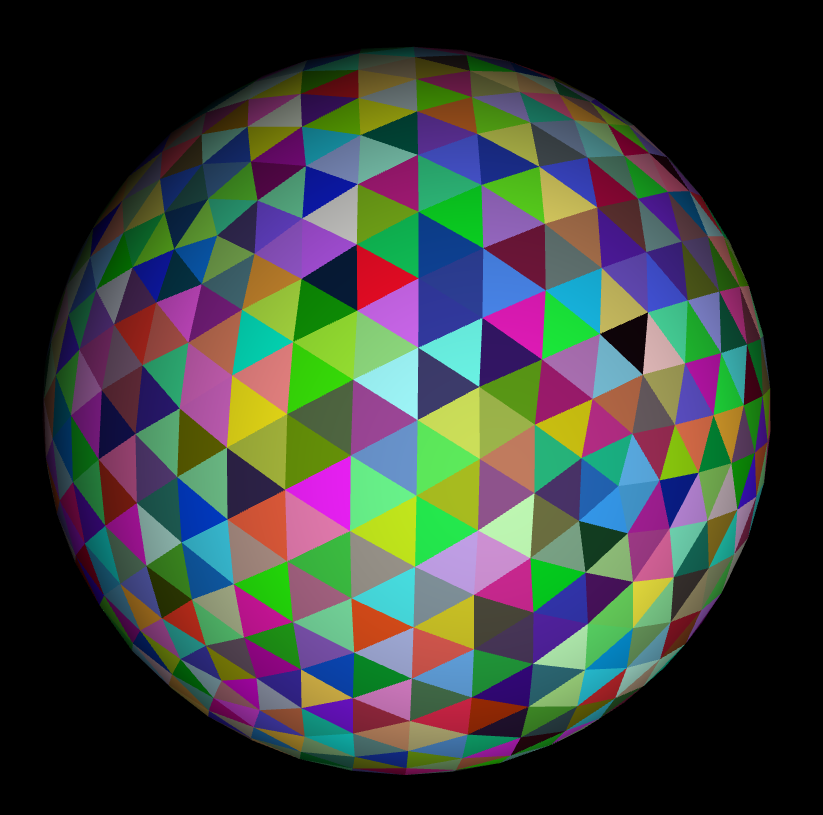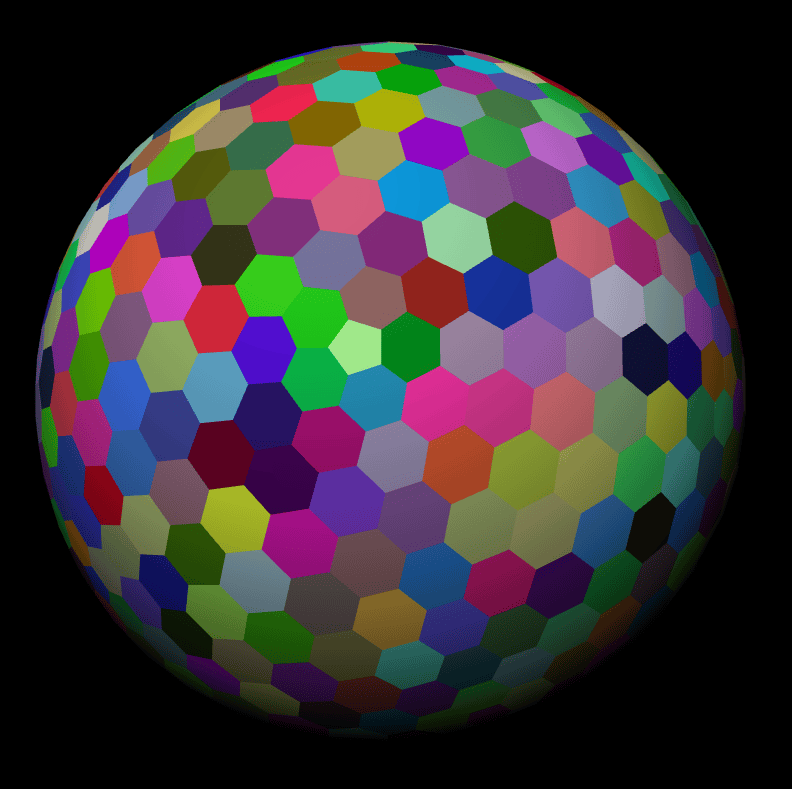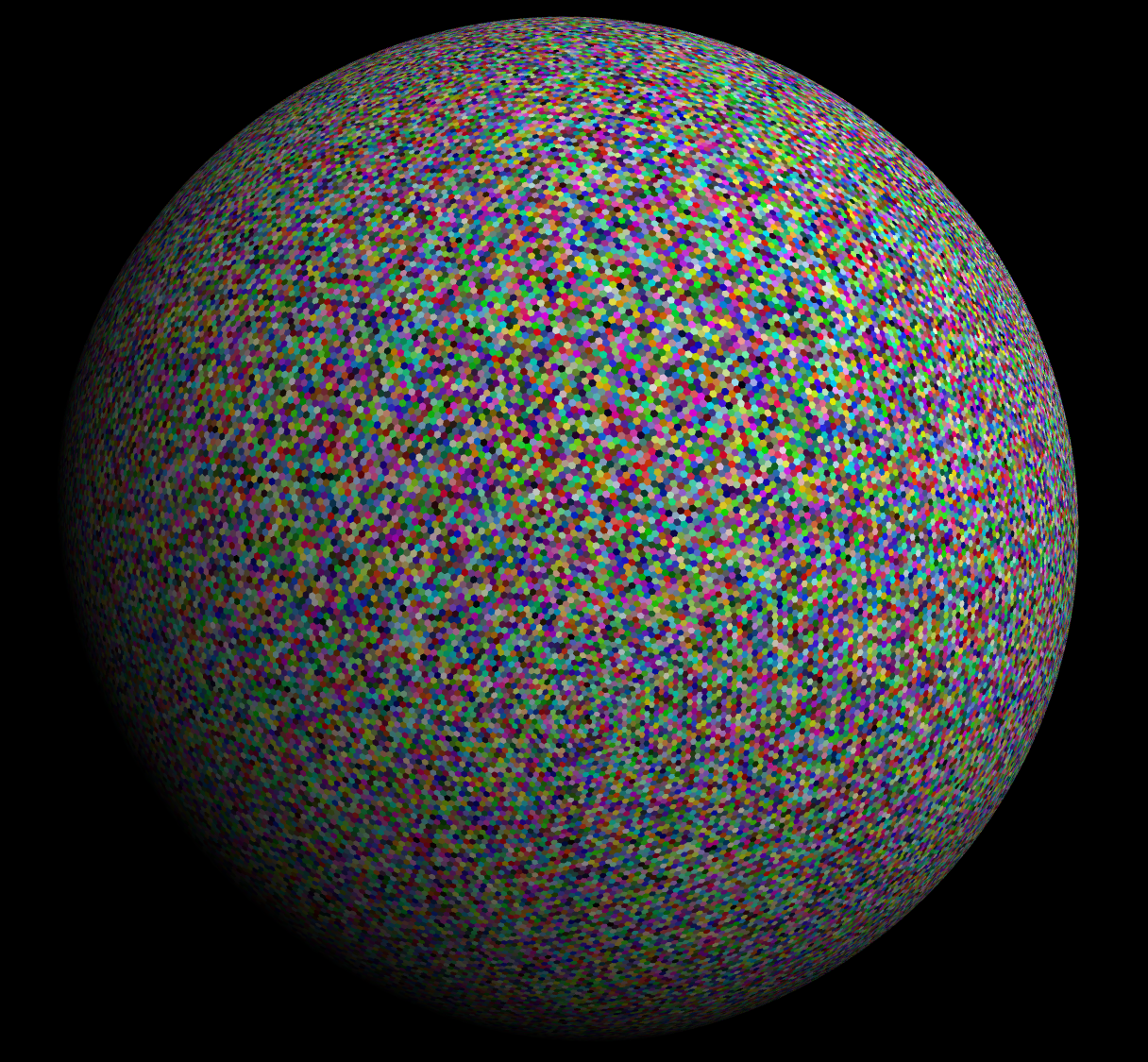## bin+lib icosahedron

Generates subdivided and truncated icosahedron spheres

### 2 releases

✓ Uses Rust 2018 edition

 0.1.1 Jul 5, 2019 Jul 5, 2019

#18 in Data formats

2MB
660 lines

Generates the shapes and then serializes them to a file with a list of vertices (`positions`) and a list of triangle faces (`cells`) that index into the list of vertices. Suitable for input into Three.js's BufferGeometry or regl.

Icosahedrons can be generated significantly faster than Three.js's version in JavaScript (which I pretty much copied into Rust).

Trunacated icosahedrons (I call them hexspheres) are a bit slower to generate since they are made by generating a icosahedron and then truncating every point into hexagon and pentagon faces.

The program can also add color to each face by assigning each vertex a color, but this comes at the cost of duplicating the shared vertices in the base model so each face has a unique set of vertices, greatly increasing the size of the mesh.

When rendering hexspheres of detail level 5 and higher and icosahedrons of detail level of 7 and higher in WebGL, make sure to enable the `OES_element_index_uint` extension since the number of vertices might overflow the normal int index.

## Screenshots

To generate a detailed hexsphere, it starts with base icosahedron:Subdivides its sides into a more detailed icosahedron:Then, truncates every point in the icosahedron into hexagons and 12 pentagons:Up to any detail level:## Install

To install, either run `cargo install icosahedron` or checkout the repo and run `cargo build --release`.

## Usage

Run it with the following options:

``````icosahedron 0.1.1
Generates 3D icosahedra meshes

USAGE:
icosahedron [FLAGS] [OPTIONS] [OUTPUT]

FLAGS:
-c, --colored      Assigns a random color to every face (increases vertices count).
-h, --help         Prints help information
-t, --truncated    Generate truncated icosahedra (hexspheres).
-V, --version      Prints version information

OPTIONS:
-d, --detail <detail>    Maximum detail level to generate. Each level multiplies the number of triangles by 4.
[default: 7]
-f, --format <format>    Format to write the files in. [default: Bin]  [possible values: Json, Bin]

ARGS:
<OUTPUT>    Directory to write the output files to. [default: output/]
``````

## Output Format

Outputs in either JSON or custom binary format. The binary format (all little endian) is laid out as:

1. 1 32 bit unsigned integer specifying the number of vertices (`V`)
2. 1 32 bit unsigned integer specifying the number of triangles (`T`)
3. `V` * 3 number of 32 bit floats for every vertex's x, y, and z coordinate
4. `V` * 3 number of 32 bit floats for the normals of every vertex
5. `V` * 3 number of 32 bit floats for the color of every vertex
6. `T` * 3 number of 32 bit unsigned integers for the 3 indices into the vertex array that make every triangle

An example of reading the binary format in JavaScript:

``````fetch(binaryFile)
.then(response => response.arrayBuffer())
.then(buffer => {